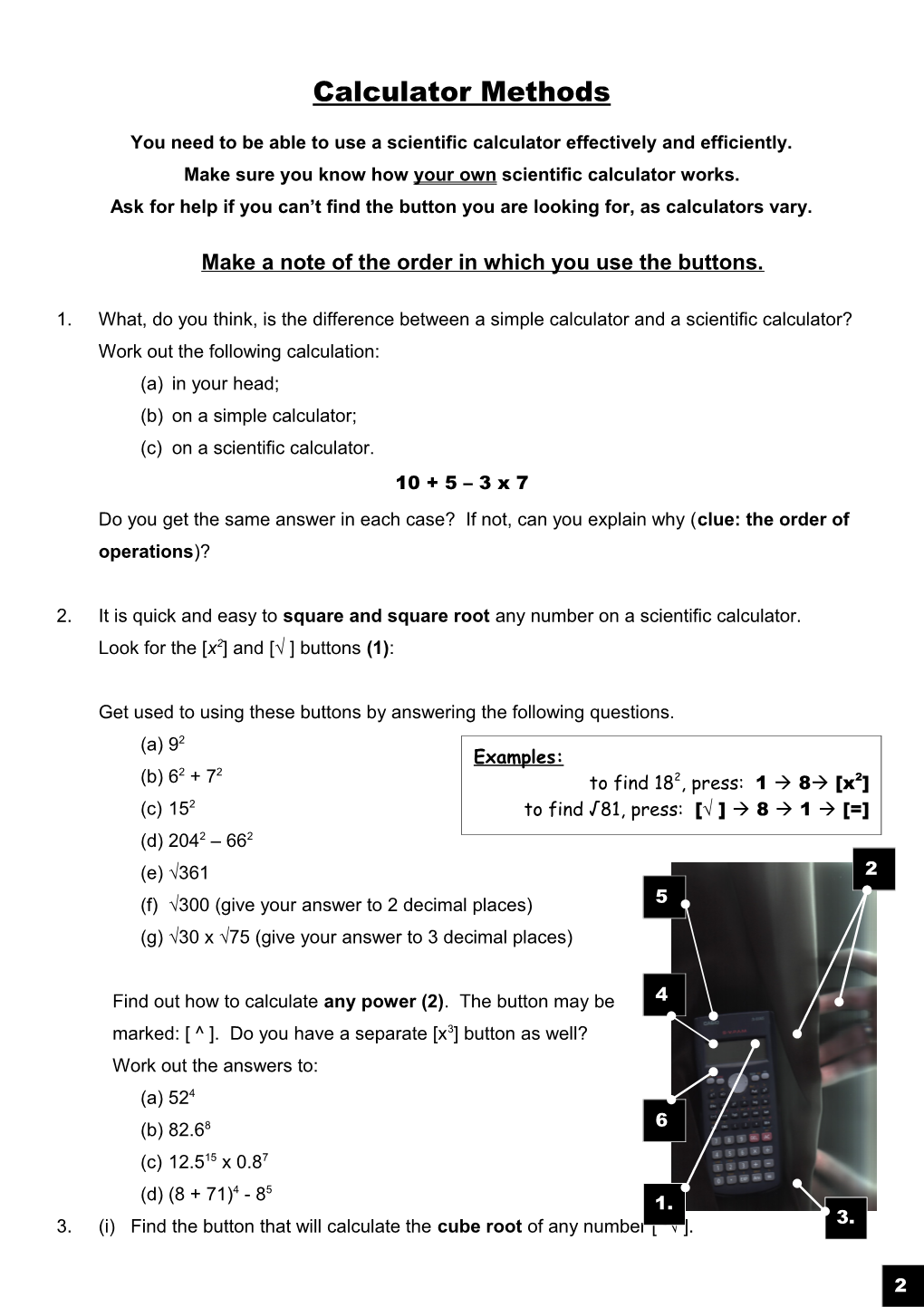# You Need to Be Able to Use a Scientific Calculator Effectively and EfficientlyCalculator Methods

You need to be able to use a scientific calculator effectively and efficiently.

Make sure you know how your own scientific calculator works.

Ask for help if you can’t find the button you are looking for, as calculators vary.

Make a note of the order in which you use the buttons.

1. What, do you think, is the difference between a simple calculator and a scientific calculator?

Work out the following calculation:

(b)on a simple calculator;

(c)on a scientific calculator.

10 + 5 – 3 x 7

Do you get the same answer in each case? If not, can you explain why (clue: the order of operations)?

1. It is quick and easy to square and square rootany number on a scientific calculator.

Look for the [x2] and [√ ] buttons(1):

Get used to using these buttons by answering the following questions.

(a)92

(b)62 + 72

(c)152

(d)2042 – 662

(e)√361

Find out how to calculate anypower (2). The button may be

marked: [ ^ ]. Do you have a separate [x3] button as well?

(a)524

(b)82.68

(c)12.515 x 0.87

(d)(8 + 71)4 - 85

1. (i) Find the button that will calculate the cube root of any number [ 3√ ].

What is the cube root of:

(a) 729(b) 3375(c) 21.952(d) 195112?

(ii) How do you work out:

(a) 6√729 (b) 9√19683?

1. Find where the pi [π]button is (3).

Write down the value of pi as it is displayed on your calculator.

Use this exact value of pi to work out the area of the following circles…

(a)(b)

1.2m

0.2mm

5.Find the fractions button (4). It probably looks like [a b/c].

If you use the [SHIFT] button, you can use the [d/c] function.

Enter the following into your calculator: 2 [a b/c] 1 [a b/c] 3 [a b/c]

This will look a bit like:

2 ┘ 1 ┘ 3

on the screen, and it is the calculator’s way of showing .

Your calculator will give you the answer in terms of a mixed number, but you can convert this into a top-heavy fraction. Without clearing 2 ┘ 1 ┘3 just press [SHIFT] [a b/c] [=]. The screen should now show 7┘3, which means .

Write down the answers to these questions as (a) a mixed number;

(b) a top-heavy fraction:

(i) (ii) (iii)

6. The reciprocal function (5) is a button that looks like [x-1] or [1/x]. This will turn a fraction upside down!

Work out these division questions by finding the reciprocal of the second fraction, then multiplying your answer by the first fraction…

(a)

(b)

(c)

7. To enter negative numbers you need to locate the [-] key (6).

Use this button to work out:

(a) -8 + - 13 + 9(b) -7 - - 20 + 6(c) 14 - - 14 + 22 – 9

8.Work out 0.0005 ÷ 10000 on your calculator.

The display probably looks a bit like this:

5.-08

…which is a standard form answer, which means:

5.0 x 10-8

Remember to write out standard form answers properly, and don’t just copy from your calculator!

Work out the following, writing down your answers in correct standard form:

(a)1000000000 x 56

(b)69.35 ÷ 10000000

(c)3.6978 x 12345678900

(d)0.00015 ÷ 10000

9.You can enter in your own standard form numbers using the [EXP] button (3).

• To type in 5.6 x 105, press:

5  .  6  [EXP]  5

• To type in 6.97 x 10-12, press:

6  .  9  7  [EXP]  [-]  1  2

(a)(5.6 x 105) x (6.97 x 10-12)

(b)(9.56 x 10-2) x (3.0 x 10-1)

(c)(1.56 x 10-9) x (-2.3 x 106)

(d)(-5.6 x 10-2) + (6.9 x 10-2)

(e)(5.016 x 104) - (3.55 x 10-3)

(f)(5.6 x 105) ÷ (6.97 x 10-12)

(g)(3.33 x 108) ÷ (9.8 x 107)

Finished Early?

How many other buttons on your calculator do you know how to use?

Can you work the memory?

Can you generate a random number? Or a set of random numbers?

Do you know how to use the percentage button?